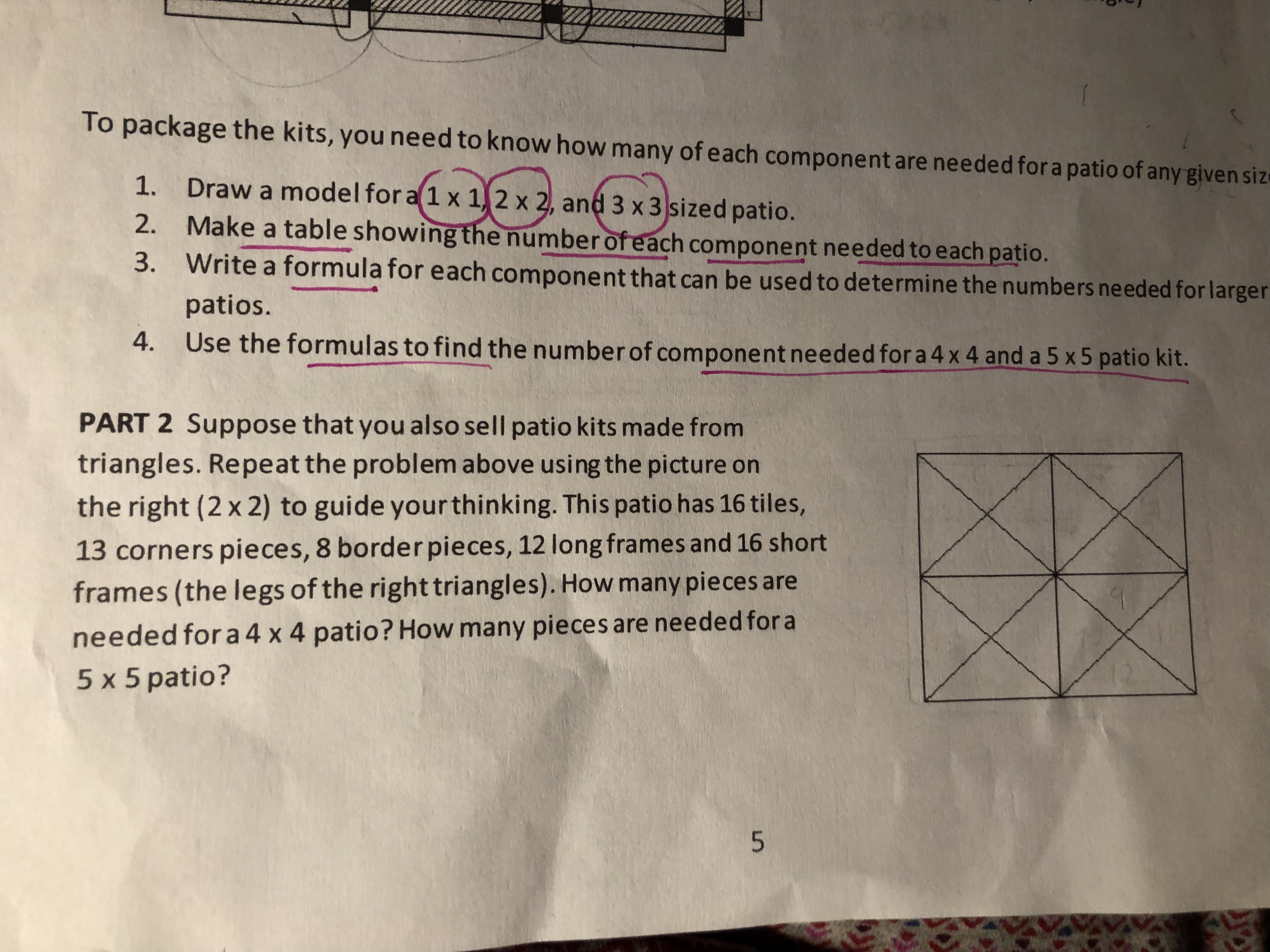# To package the kits, you need to know how many of each component are needed for a patio of any given size Draw a model for a 1 x 1,2 x 2, and 3 x 3 sized patio. Make a table showing the number of each component needed to each patio. Write a formula for each component that can be used to determine the numbers needed for larger 1. 2. 3. patios. 4. Use the formulas to find the number of component needed for a 4 x 4 and a 5 x5 patio kit. PART 2 Suppose that you also sell patio kits made from triangles. Repeat the problem above using the picture on the right (2 x 2) to guide yourthinking. This patio has 16 tiles, 13 corners pieces, 8 border pieces, 12 long frames and 16 short frames (the legs of the right triangles). How many pieces are needed for a 4 x4 patio? How many pieces are needed for a 5 x 5 patio? 5

Question

For part 2 how can i figure the formulas of the components of the kits made from triangles?help_outlineImage TranscriptioncloseTo package the kits, you need to know how many of each component are needed for a patio of any given size Draw a model for a 1 x 1,2 x 2, and 3 x 3 sized patio. Make a table showing the number of each component needed to each patio. Write a formula for each component that can be used to determine the numbers needed for larger 1. 2. 3. patios. 4. Use the formulas to find the number of component needed for a 4 x 4 and a 5 x5 patio kit. PART 2 Suppose that you also sell patio kits made from triangles. Repeat the problem above using the picture on the right (2 x 2) to guide yourthinking. This patio has 16 tiles, 13 corners pieces, 8 border pieces, 12 long frames and 16 short frames (the legs of the right triangles). How many pieces are needed for a 4 x4 patio? How many pieces are needed for a 5 x 5 patio? 5 fullscreen

### Want to see this answer and more?

Experts are waiting 24/7 to provide step-by-step solutions in as fast as 30 minutes!*

*Response times vary by subject and question complexity. Median response time is 34 minutes and may be longer for new subjects.
Tagged in
MathGeometry

### Triangles